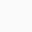# Molarity of 50% (w/w) Sodium Hydroxide (NaOH)

OVERVIEW• A 50% (w/w) concentrated Sodium hydroxide solution is a clear colorless liquid. It can be purchased from many suppliers.
• A 50% (w/w) sodium hydroxide solution means that there is 50 g of NaOH per 100 g of solution.
• The density of 50% (w/w) Sodium hydroxide solution is 1.515 g/ml at 25°C which means that the weight of the 1 ml of Sodium hydroxide solution is 1.515 g at 25°C.
• Molarity refers to the number of moles of the solute present in 1 liter of solution.
• In simple words, 1 mole is equal to the atomic weight of the substance. For example, 1 mole of Sodium hydroxide is equal to 40.00 grams of Sodium hydroxide (NaOH, molecular weight = 40.00).
• To calculate the molarity, one must first calculate how much Sodium hydroxide is present in 1 L of 50% Sodium hydroxide solution. Once we know the amount of sodium hydroxide present in 1 L solution, we can calculate the molarity of the solution by dividing the NaOH amount by the molecular weight.## Calculation procedure:

Step 1: Calculate the volume of 100 grams of Sodium Hydroxide solution.
Formula:

Density = $\frac{Weight}{Volume}$

OR

Volume = $\frac{\mathrm{Weight}}{\mathrm{Density}}$

The volume of 100 g of Sodium hydroxide solution: $\frac{00}{1.515}$  = 66.0066 ml

Note: 50% (w/w) Sodium Hydroxide means that 100 g of solution contains 50 g of Sodium Hydroxide.

The volume of 100 g of Sodium hydroxide solution is 66.0066 ml which means 50 g of NaOH is present in 66.0066 ml of  Sodium hydroxide solution.

Step 2: Calculate how many grams of NaOH is present in 1000 ml of Sodium hydroxide solution.
66.0066 ml of Sodium Hydroxide solution contains   = 50 grams of NaOH
1 ml of Sodium hydroxide solution will contain      =   $\frac{50}{66.0066}$ grams of NaOH

1000 ml of Sodium hydroxide solution will contain =     = 757.50 grams of NaOH

1000 ml of Sodium hydroxide solution will contain 757.50 grams of NaOH.

Step 3: Calculate the number of moles of NaOH present in 757.50 grams of NaOH.
40 g of NaOH is equal to 1 mole.

1 g of NaOH will be equal to $\frac{1}{40}$  moles.

Therefore, we can say that 1 liter of sodium hydroxide solution contains 18.9375 moles or in other words molarity of 50% (w/w) Sodium Hydroxide is equal to 18.9375 M.Calculator – Calculate the molarity of concentrated Sodium Hydroxide (NaOH)
Use Calculator to calculate the molarity of concentrated Sodium Hydroxide (NaOH) when concentration is given in % by mass (w/w)

Sodium Hydroxide (NaOH) Molecular weight: 40.00 g/mole

Concentration of Sodium Hydroxide in % (wt/wt) : % (wt/wt)

(Change the % (wt/wt) concentration)

Density of glacial Sodium Hydroxide: g/ml

(Change the density)

Molarity of Sodium Hydroxide: 18.938 M1.Paul says:
2.Andrew says: